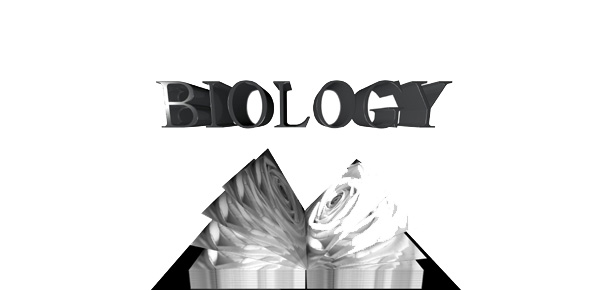# Principles Of Biology Chapters 2 & 3 ((Continued))

54 Questions | Total Attempts: 68SettingsThis is a continued version of the quiz Principles of Biology Chapter 2 & 3. I have added and re-wrote certain questions. Enjoy.

• 1.
What subatomic particle has a negative charge?
• A.

Proton

• B.

Neutron

• C.

Electron

• 2.
What subatomic particle has no charge?
• A.

Electron

• B.

Neutron

• C.

Proton

• 3.
What subatomic particle has a positive charge?
• A.

Neutron

• B.

Proton

• C.

Electron

• 4.
What are the subatomic particles?
• 5.
Which 2 subatomic particles are located inside the atom's nucleus? (multiple answers)
• A.

Proton

• B.

Electron

• C.

Neutron

• 6.
Which subatomic particle is located orbiting outside of the atom's nucleus?
• A.

Proton

• B.

Neutron

• C.

Electron

• 7.
Which subatomic particle is involved with forming chemical bonds?
• A.

Proton

• B.

Neutron

• C.

Electron

• 8.
What are the levels of organization of living things?Hint: AMCTOOOPCEB
• 9.
What are the levels of classification of living things? (List them in order)Hint: What does King Philip do?
• 10.
What is the term for atoms that are electrically charged as a result of gaining or losing electrons?
• 11.
Which is the reactant?Ex.A+B ---> C
• A.

A

• B.

B

• C.

A+B

• D.

C

• 12.
Which is the product?Ex.A+B ---> C
• A.

A+B

• B.

B

• C.

A

• D.

C

• 13.
What reaction is the process of smaller things built up into larger?Ex.A+B ---> C(Sm) (L)
• A.

Anabolic

• B.

Catabolic

• 14.
What reaction is the process of larger things breaking down to smaller?Ex.C ---> A+B(L) (Sm)
• A.

Anabolic

• B.

Catabolic

• 15.
The ______ bond holds one water molecule to another water molecule.
• 16.
What does water do when is freezes?
• A.

Expand

• B.

Condense

• 17.
What are the properties of water?(multiple answers)
• A.

Cohesion

• B.

Surface tension

• C.

Temperature regulation

• D.

Freezing properties

• E.

Solvent for biochemical reactions

• 18.
The fluids of organisms are ______ solutions.
• 19.
A ___ _____ is used to measure the hydrogen ion (H+) concentration in a solution.
• 20.
What is the chemical compound that releases hydrogen ions (H+) to a solution?Hint: below 7.0 on the pH scale
• A.

Acids

• B.

Bases

• C.

Buffers

• 21.
What is the chemical compound that accepts hydrogen ions (H+) and removes it from a solution?Hint: above 7.0 on the pH scale
• A.

Acids

• B.

Bases

• C.

Buffers

• 22.
What pH level makes a solution chemically neutral?
• A.

Above 7.0

• B.

Below 7.0

• C.

7.0

• 23.
What does HONC stand for?
• 24.
Hydrogen has ___ bond(s).
• 25.
Carbon has ___ bond(s).Back to top